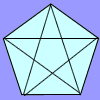#### You may also like### Number Detective

Follow the clues to find the mystery number.### Prime Magic

Place the numbers 1, 2, 3,..., 9 one on each square of a 3 by 3 grid so that all the rows and columns add up to a prime number. How many different solutions can you find?### Diagonal Trace

You can trace over all of the diagonals of a pentagon without lifting your pencil and without going over any more than once. Can the same thing be done with a hexagon or with a heptagon?

# Red Even

##### Age 7 to 11 Challenge Level:

You have 4 even numbers and 5 odd numbers and all the totals are odd. Think of the red counters as even numbers and the blues as odds. If diagonals can have any total then all three configurations give equivalent solutions because one can be transformed into another by interchanging rows or interchanging columns. If diagonals also have prime totals then only configuration (a) is possible